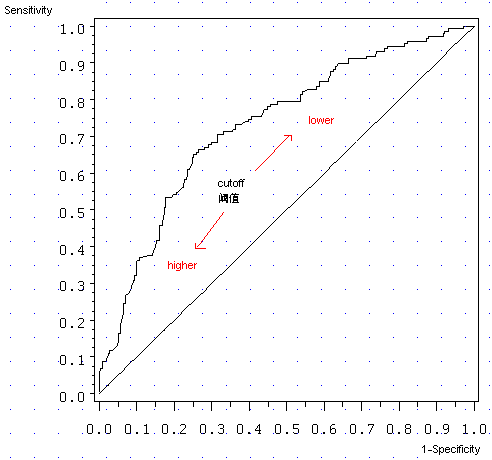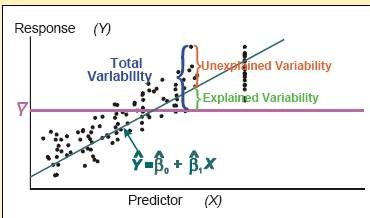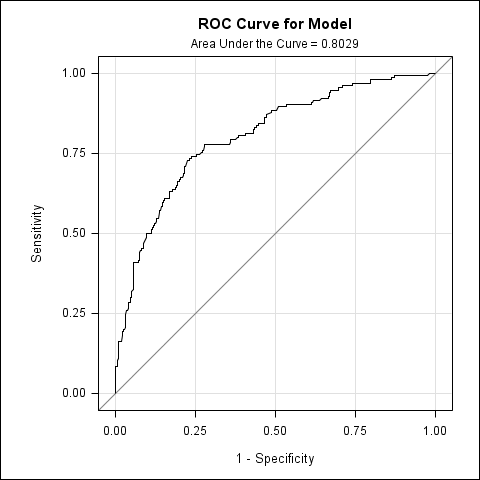# ROC

Sensitivity（覆盖率，True Positive Rate）

1-Specificity (Specificity, 负例的覆盖率，True Negative Rate)

axis order=(0 to 1 by .1) label=none length=4in;

symbol i=join v=none c=black;

symbol2 i=join v=none c=black;

proc gplot data = valid_roc;

plot _SENSIT_*_1MSPEC_ _1MSPEC_*_1MSPEC_

/ overlay vaxis=axis haxis=axis;

run; quit;1 0
1 d, True Positive c, False Negative c+d, Actual Positive
0 b, False Positive a, True Negative a+b, Actual Negative
b+d, Predicted Positive a+c, Predicted Negative# AUC, Area Under the ROC Curve

ROC曲线是根据与45度线的偏离来判断模型好坏。图示的好处是直观，不足就是不够精确。到底好在哪里，好了多少？这就要涉及另一个术语，AUC(Area Under the ROC Curve，ROC曲线下的面积)，不过也不是新东西，只是ROC的一个派生而已。

SAS的Logistic回归能够后直接生成AUC值。跑完上面的模型，你可以在结果报告的Association Statistics找到一个叫c的指标，它就是AUC（本例中，c=AUC=0.803，45度线的c=0.5）。

# ROC、AUC：SAS9.2一步到位

SAS9.2有个非常好的新功能，叫ODS Statistical Graphics，有兴趣可以去它主页看看。在SAS9.2平台提交以下代码，Logistic回归参数估计和ROC曲线、AUC值等结果就能一起出来（有了上面的铺垫，就不惧这个黑箱了）：

ods graphics on;

proc logistic data=train plots(only)=roc;

model good_bad=checking history duration savings property;

run;

ods graphics off;# 参考资料

1. Mithat Gonen. 2007. Analyzing Receiver Operating Characteristic Curves with SAS. Cary, NC: SAS Institute Inc.
2. Mike Patetta. 2008. Categorical Data Analysis Using Logistic Regression Course Notes. Cary, NC: SAS Institute Inc.
3. Dan Kelly, etc. 2007. Predictive Modeling Using Logistic Regression Course Notes. Cary, NC: SAS Institute Inc.